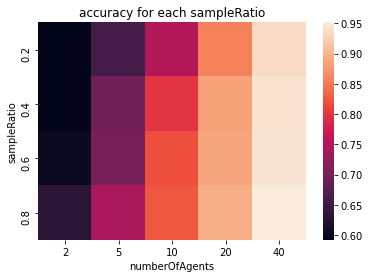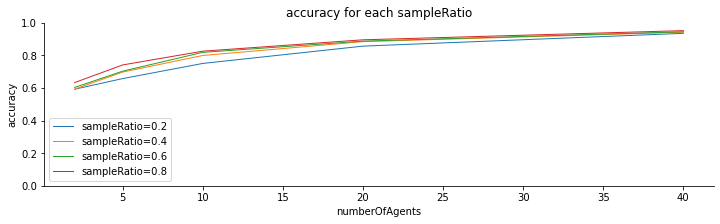# Experiment 20200623-DOLA¶

## Experiment design¶

Agent adapt ontologies to agree on decision taking

Date: 20200623 (Yasser Bourahla)

Hypothesis: ['Success rate converges to 1', ' Improve the average accuracy at the end of the experiment', ' Agents do not necessarily converge to the same ontology', '']

10 runs; 40000 games

Experimental setting: Agents learn decision trees (transformed into ontologies); get income from environment; adapt by splitting their leaf nodes

#### Variables¶

independent variables: ['numberOfAgents', 'numberOfFeatures', 'numberOfClasses', 'ratio', 'sampleRatio']

dependent variables: ['ssrate', 'accuracy', 'distance']

## Experiment¶

Date: 20200623 (Yasser Bourahla)

LazyLavender hash: 4b837b2619086143a5bbb798ac0b80b110f80342

Parameter file: params.sh

Executed command (script.sh):

BEWARE: REPRODUCING THE ANALYSIS TAKES A CONSIDERABLE AMOUNT OF TIME.

#!/bin/bash

. params.sh

CURRDIR=$(pwd) OUTPUT=${CURRDIR}/${DIRPREF} # cd${LLPATH}
cd lazylav
# this sample runs ExperimentalPlan. It can be replaced with Monitor if parameters are not varied.
bash scripts/runexp.sh -p ${CURRDIR} -d${DIRPREF} java -Dlog.level=INFO -cp ${JPATH} fr.inria.exmo.lazylavender.engine.ExperimentalPlan -Dexperiment=fr.inria.exmo.lazylavender.decisiontaking.Experiment${OPT} -DresultDir=\${OUTPUT}


## Experimental plan¶

The independent variables have been varied as follows:

number of agents: [2, 5, 10, 20, 40]
number of features: [3, 4, 5]
number of classes: [2, 3, 4]
training ratio: [0.1, 0.3, 0.5]
task ratio: [0.2, 0.4, 0.6, 0.8]

## Raw results¶

Full results are available on zenodo

## Statistical Analysis¶

Three hypotheses are tested:

• hypothesis 1: the success rate (ssrate) will converge to 1.
• hypothesis 3: the average accuracy at the end of the experiment is significantly different (higher) than that of at the beginning of the experiment
• hypothesis 2: final distance is different from 0 most of the time.

## Hypothesis 1:¶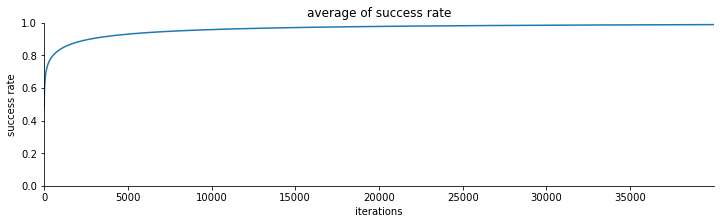hypothesis 1 verified


## Hypothesis 2¶

We check if there is a significant difference between the starting average accuracy and the final average accuracy.

There is a significant difference in the accuracy between the start and the end of the experiment.
Paired t-test results: t=-100.06 and p<0.01.
Hypothesis 2 verified.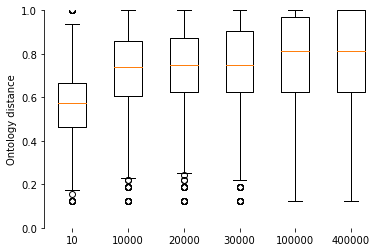## Hypothesis 3¶

the percentage of final none zero distances to all distances is : 90.78 %
hypothesis 3 verified.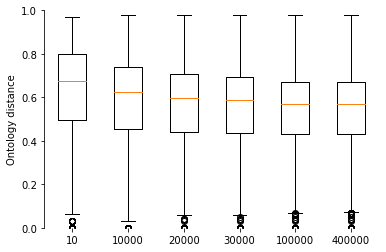proportion of runs having end accuracy lower than start accuracy to all runs is 0.035.

Table contains number of runs in which the accuracy dropped by each factor value and its proportion to all runs having that value:

number of agents number of features number of classes training ratio task ratio
2 5 10 20 40 3 4 5 2 3 4 0.1 0.3 0.5 0.2 0.4 0.6 0.8
nb runs drop 141 44 4 0 0 50 74 65 49 64 76 57 61 71 84 52 40 13
proportion to rest 0.04 0.01 0.00 0.00 0.00 0.01 0.01 0.01 0.01 0.01 0.01 0.01 0.01 0.01 0.02 0.01 0.01 0.00
number of runs in which accuracy drops by number of agents(columns) and task ratio(rows):

2 5 10 20 40
0.20 53 29 2 0 0
0.40 43 9 0 0 0
0.60 32 6 2 0 0
0.80 13 0 0 0 0

# Data exploration¶

Summary of results by factor values

number of agents number of features number of classes training ratio task ratio
2 5 10 20 40 3 4 5 2 3 4 0.1 0.3 0.5 0.2 0.4 0.6 0.8
ssrate 1 0.47 0.48 0.51 0.46 0.47 0.50 0.47 0.47 0.58 0.48 0.37 0.43 0.47 0.54 0.47 0.47 0.48 0.50
10000 1.00 0.96 0.86 0.74 0.65 0.91 0.84 0.77 0.89 0.83 0.80 0.87 0.81 0.84 0.82 0.83 0.85 0.86
20000 1.00 0.98 0.91 0.81 0.71 0.94 0.89 0.81 0.92 0.88 0.85 0.90 0.86 0.88 0.86 0.88 0.89 0.90
30000 1.00 0.99 0.94 0.85 0.75 0.96 0.91 0.84 0.94 0.90 0.88 0.92 0.89 0.91 0.88 0.90 0.91 0.92
100000 1.00 1.00 0.98 0.94 0.87 0.99 0.97 0.92 0.97 0.96 0.94 0.96 0.95 0.96 0.94 0.96 0.96 0.97
400000 1.00 1.00 1.00 0.99 0.96 1.00 0.99 0.98 0.99 0.99 0.98 0.99 0.99 0.99 0.98 0.99 0.99 0.99
accuracy 1 0.57 0.56 0.56 0.56 0.56 0.58 0.56 0.56 0.66 0.54 0.49 0.45 0.56 0.68 0.56 0.56 0.56 0.57
10000 0.61 0.70 0.77 0.77 0.73 0.76 0.72 0.66 0.79 0.70 0.65 0.58 0.73 0.83 0.67 0.71 0.72 0.75
20000 0.61 0.70 0.79 0.82 0.79 0.78 0.75 0.70 0.81 0.73 0.69 0.59 0.77 0.86 0.70 0.74 0.75 0.78
30000 0.61 0.70 0.80 0.84 0.83 0.78 0.76 0.72 0.82 0.74 0.70 0.61 0.78 0.87 0.71 0.75 0.77 0.79
100000 0.61 0.70 0.80 0.88 0.92 0.79 0.78 0.77 0.84 0.77 0.74 0.63 0.82 0.90 0.75 0.78 0.79 0.81
400000 0.61 0.70 0.80 0.88 0.94 0.79 0.78 0.79 0.84 0.77 0.74 0.64 0.82 0.90 0.76 0.78 0.79 0.81
distance 1 0.56 0.61 0.62 0.62 0.61 0.43 0.61 0.77 0.58 0.61 0.62 0.39 0.69 0.73 0.61 0.60 0.60 0.60
10000 0.47 0.47 0.48 0.55 0.65 0.34 0.53 0.70 0.54 0.52 0.50 0.41 0.58 0.58 0.52 0.52 0.53 0.52
20000 0.47 0.47 0.47 0.49 0.57 0.33 0.50 0.65 0.52 0.49 0.47 0.38 0.55 0.55 0.49 0.49 0.50 0.50
30000 0.47 0.47 0.47 0.48 0.53 0.33 0.49 0.63 0.51 0.48 0.46 0.37 0.53 0.54 0.47 0.48 0.49 0.49
100000 0.47 0.47 0.47 0.47 0.48 0.33 0.49 0.60 0.50 0.47 0.44 0.35 0.52 0.54 0.46 0.47 0.48 0.48
400000 0.47 0.47 0.47 0.47 0.48 0.33 0.49 0.60 0.50 0.47 0.44 0.35 0.52 0.54 0.46 0.47 0.48 0.48

### Statistical analysis¶

ANOVA is applied to determine which factors significantly influence which measures

influence of number of agents
PR(>F) Significance
ssrate 0.000000 True
accuracy 0.000000 True
distance 0.475119 False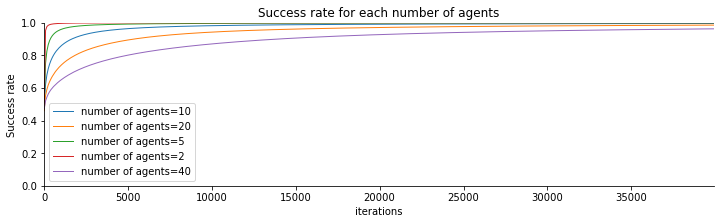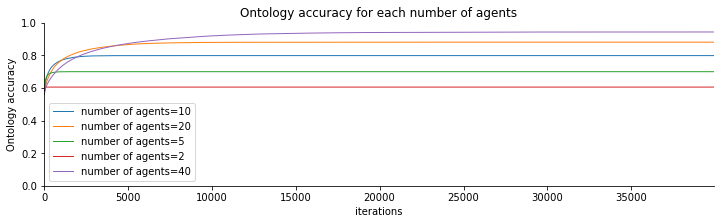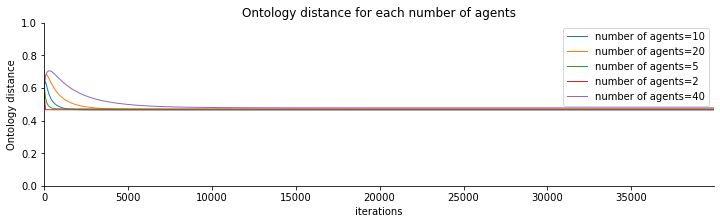influence of number of features
PR(>F) Significance
ssrate 0.000000 True
accuracy 0.407979 False
distance 0.000000 True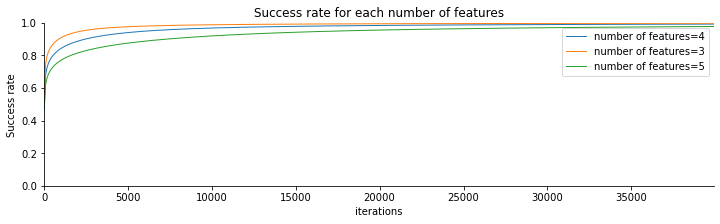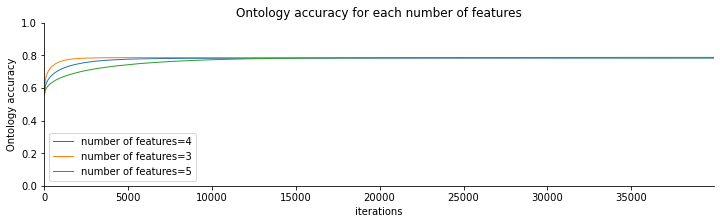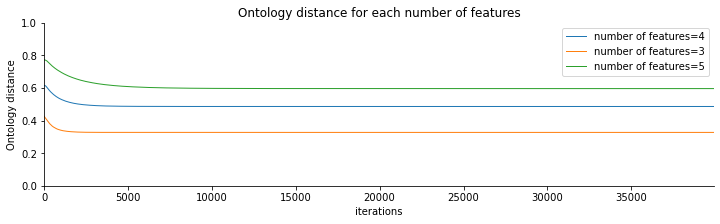influence of number of classes
PR(>F) Significance
ssrate 0.000000 True
accuracy 0.000000 True
distance 0.000000 True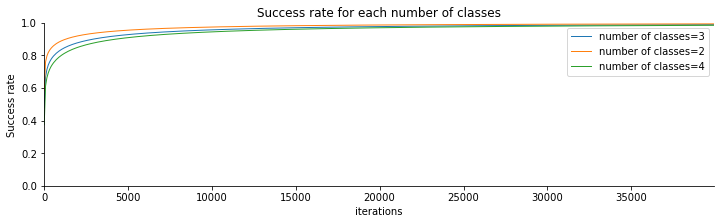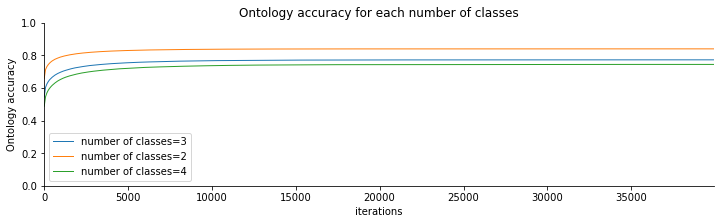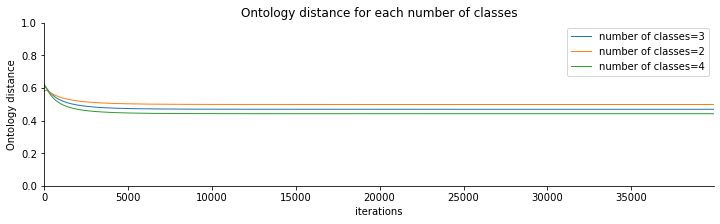influence of training ratio
PR(>F) Significance
ssrate 0.000000 True
accuracy 0.000000 True
distance 0.000000 True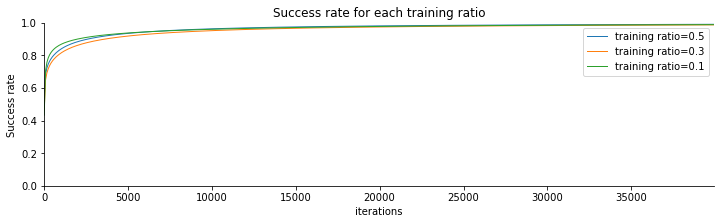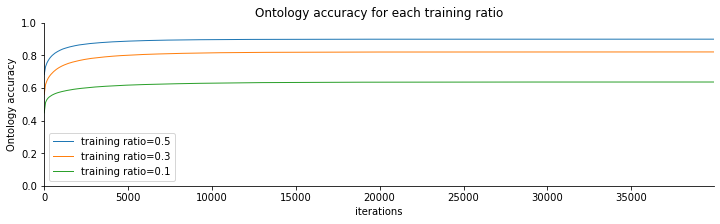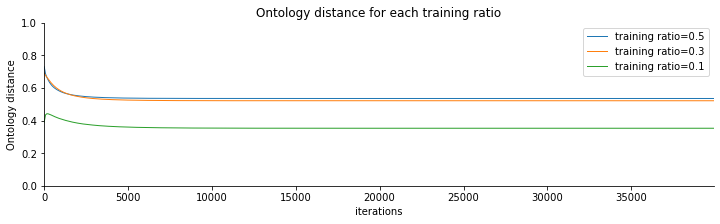PR(>F) Significance
ssrate 0.000000 True
accuracy 0.000000 True
distance 0.002343 True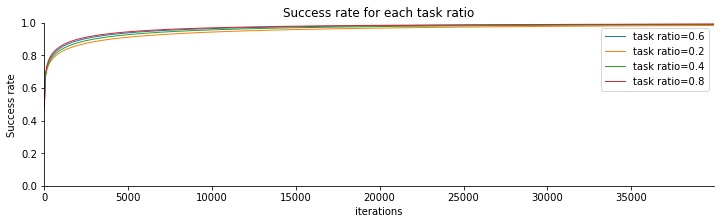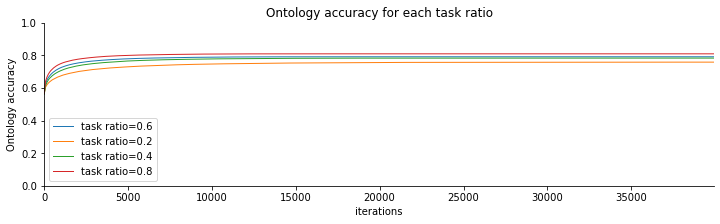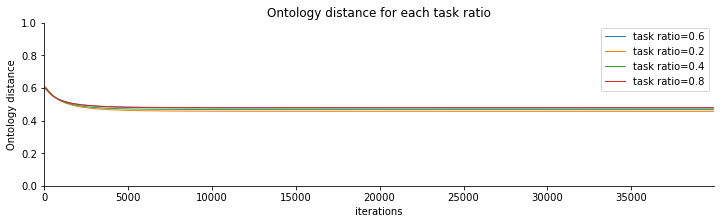## Full ANOVA and Post-hoc tables¶

For each dependent variable: Print it's ANOVA table (1) and for each independent variable: print it's post-hoc test table using the Tukey-hsd (honestly significant difference) test.

#### ANOVA table¶

ANOVA table is organised in columns where each column concerns one independent variable as follows:

Parameter df sum_sq mean_sq F PR(>F)
independent variable Degrees of freedom Sum of squares sum_sq/df F statistic value P-value

#### Post-hoc table¶

For each independent variable a post-hoc table is printed. Post-hoc table is also organised in columns. Each column contains the comparison of two possible values of the independent variable.

group 1 group 2 mean-diff p-adj lower upper reject
Possible value of the independent variable A different possible value of the independent variable pairwise mean difference adjusted p-value lower bound of confidence interval for pairwise mean differences upper bound of confidence interval for pairwise mean differences p-adj < 0.05
***********************************************************
FULL STATISTICAL RESULTS
***********************************************************
***********************************************************
ANOVA results for ssrate
***********************************************************

df sum_sq mean_sq F PR(>F)
C(Q("numberOfAgents")) 4.00 1.05 0.26 6,900.48 0.00
C(Q("numberOfFeatures")) 2.00 0.38 0.19 5,051.74 0.00
C(Q("numberOfClasses")) 2.00 0.07 0.03 916.55 0.00
C(Q("ratio")) 2.00 0.01 0.01 133.69 0.00
C(Q("sampleRatio")) 3.00 0.06 0.02 552.26 0.00
C(Q("numberOfAgents")):C(Q("numberOfFeatures")) 8.00 0.58 0.07 1,901.96 0.00
C(Q("numberOfAgents")):C(Q("numberOfClasses")) 8.00 0.11 0.01 361.79 0.00
C(Q("numberOfFeatures")):C(Q("numberOfClasses")) 4.00 0.04 0.01 276.87 0.00
C(Q("numberOfAgents")):C(Q("ratio")) 8.00 0.04 0.00 125.49 0.00
C(Q("numberOfFeatures")):C(Q("ratio")) 4.00 0.01 0.00 53.76 0.00
C(Q("numberOfClasses")):C(Q("ratio")) 4.00 0.01 0.00 33.20 0.00
C(Q("numberOfAgents")):C(Q("sampleRatio")) 12.00 0.12 0.01 263.73 0.00
C(Q("numberOfFeatures")):C(Q("sampleRatio")) 6.00 0.03 0.01 135.26 0.00
C(Q("numberOfClasses")):C(Q("sampleRatio")) 6.00 0.01 0.00 22.99 0.00
C(Q("ratio")):C(Q("sampleRatio")) 6.00 0.00 0.00 3.64 0.00
C(Q("numberOfAgents")):C(Q("numberOfFeatures")):C(Q("numberOfClasses")) 16.00 0.07 0.00 109.28 0.00
C(Q("numberOfAgents")):C(Q("numberOfFeatures")):C(Q("ratio")) 16.00 0.02 0.00 41.14 0.00
C(Q("numberOfAgents")):C(Q("numberOfClasses")):C(Q("ratio")) 16.00 0.01 0.00 21.83 0.00
C(Q("numberOfFeatures")):C(Q("numberOfClasses")):C(Q("ratio")) 8.00 0.00 0.00 11.38 0.00
C(Q("numberOfAgents")):C(Q("numberOfFeatures")):C(Q("sampleRatio")) 24.00 0.06 0.00 70.74 0.00
C(Q("numberOfAgents")):C(Q("numberOfClasses")):C(Q("sampleRatio")) 24.00 0.01 0.00 11.10 0.00
C(Q("numberOfFeatures")):C(Q("numberOfClasses")):C(Q("sampleRatio")) 12.00 0.00 0.00 6.68 0.00
C(Q("numberOfAgents")):C(Q("ratio")):C(Q("sampleRatio")) 24.00 0.01 0.00 7.61 0.00
C(Q("numberOfFeatures")):C(Q("ratio")):C(Q("sampleRatio")) 12.00 0.00 0.00 5.33 0.00
C(Q("numberOfClasses")):C(Q("ratio")):C(Q("sampleRatio")) 12.00 0.00 0.00 2.52 0.00
C(Q("numberOfAgents")):C(Q("numberOfFeatures")):C(Q("numberOfClasses")):C(Q("ratio")) 32.00 0.01 0.00 6.32 0.00
C(Q("numberOfAgents")):C(Q("numberOfFeatures")):C(Q("numberOfClasses")):C(Q("sampleRatio")) 48.00 0.01 0.00 3.94 0.00
C(Q("numberOfAgents")):C(Q("numberOfFeatures")):C(Q("ratio")):C(Q("sampleRatio")) 48.00 0.01 0.00 5.40 0.00
C(Q("numberOfAgents")):C(Q("numberOfClasses")):C(Q("ratio")):C(Q("sampleRatio")) 48.00 0.00 0.00 2.07 0.00
C(Q("numberOfFeatures")):C(Q("numberOfClasses")):C(Q("ratio")):C(Q("sampleRatio")) 24.00 0.00 0.00 2.29 0.00
C(Q("numberOfAgents")):C(Q("numberOfFeatures")):C(Q("numberOfClasses")):C(Q("ratio")):C(Q("sampleRatio")) 96.00 0.01 0.00 2.02 0.00
Residual 4,860.00 0.18 0.00 nan nan
--------------------------------------
post-hoc (Tukey) test for numberOfAgents on ssrate
--------------------------------------

group1 group2 meandiff p-adj lower upper reject
0 10 2 0.00 0.00 0.00 0.01 True
1 10 20 -0.01 0.00 -0.01 -0.01 True
2 10 40 -0.03 0.00 -0.04 -0.03 True
3 10 5 0.00 0.00 0.00 0.01 True
4 2 20 -0.01 0.00 -0.02 -0.01 True
5 2 40 -0.04 0.00 -0.04 -0.04 True
6 2 5 -0.00 0.74 -0.00 0.00 False
7 20 40 -0.02 0.00 -0.02 -0.02 True
8 20 5 0.01 0.00 0.01 0.02 True
9 40 5 0.04 0.00 0.03 0.04 True
--------------------------------------
post-hoc (Tukey) test for numberOfFeatures on ssrate
--------------------------------------

group1 group2 meandiff p-adj lower upper reject
0 3 4 -0.01 0.00 -0.01 -0.00 True
1 3 5 -0.02 0.00 -0.02 -0.02 True
2 4 5 -0.01 0.00 -0.02 -0.01 True
--------------------------------------
post-hoc (Tukey) test for numberOfClasses on ssrate
--------------------------------------

group1 group2 meandiff p-adj lower upper reject
0 2 3 -0.01 0.00 -0.01 -0.00 True
1 2 4 -0.01 0.00 -0.01 -0.01 True
2 3 4 -0.00 0.00 -0.01 -0.00 True
--------------------------------------
post-hoc (Tukey) test for ratio on ssrate
--------------------------------------

group1 group2 meandiff p-adj lower upper reject
0 0.1 0.3 -0.00 0.23 -0.00 0.00 False
1 0.1 0.5 0.00 0.02 0.00 0.00 True
2 0.3 0.5 0.00 0.00 0.00 0.01 True
--------------------------------------
post-hoc (Tukey) test for sampleRatio on ssrate
--------------------------------------

group1 group2 meandiff p-adj lower upper reject
0 0.2 0.4 0.01 0.00 0.00 0.01 True
1 0.2 0.6 0.01 0.00 0.01 0.01 True
2 0.2 0.8 0.01 0.00 0.01 0.01 True
3 0.4 0.6 0.00 0.02 0.00 0.00 True
4 0.4 0.8 0.00 0.00 0.00 0.01 True
5 0.6 0.8 0.00 0.51 -0.00 0.00 False
***********************************************************
ANOVA results for accuracy
***********************************************************

df sum_sq mean_sq F PR(>F)
C(Q("numberOfAgents")) 4.00 79.84 19.96 2,419.34 0.00
C(Q("numberOfFeatures")) 2.00 0.02 0.01 1.16 0.31
C(Q("numberOfClasses")) 2.00 8.66 4.33 524.74 0.00
C(Q("ratio")) 2.00 65.50 32.75 3,969.63 0.00
C(Q("sampleRatio")) 3.00 1.81 0.60 73.28 0.00
C(Q("numberOfAgents")):C(Q("numberOfFeatures")) 8.00 0.94 0.12 14.18 0.00
C(Q("numberOfAgents")):C(Q("numberOfClasses")) 8.00 2.93 0.37 44.36 0.00
C(Q("numberOfFeatures")):C(Q("numberOfClasses")) 4.00 0.00 0.00 0.14 0.97
C(Q("numberOfAgents")):C(Q("ratio")) 8.00 4.35 0.54 65.88 0.00
C(Q("numberOfFeatures")):C(Q("ratio")) 4.00 0.79 0.20 23.87 0.00
C(Q("numberOfClasses")):C(Q("ratio")) 4.00 2.14 0.53 64.71 0.00
C(Q("numberOfAgents")):C(Q("sampleRatio")) 12.00 0.63 0.05 6.34 0.00
C(Q("numberOfFeatures")):C(Q("sampleRatio")) 6.00 0.09 0.02 1.90 0.08
C(Q("numberOfClasses")):C(Q("sampleRatio")) 6.00 0.26 0.04 5.18 0.00
C(Q("ratio")):C(Q("sampleRatio")) 6.00 0.21 0.03 4.23 0.00
C(Q("numberOfAgents")):C(Q("numberOfFeatures")):C(Q("numberOfClasses")) 16.00 0.11 0.01 0.85 0.63
C(Q("numberOfAgents")):C(Q("numberOfFeatures")):C(Q("ratio")) 16.00 0.85 0.05 6.43 0.00
C(Q("numberOfAgents")):C(Q("numberOfClasses")):C(Q("ratio")) 16.00 0.22 0.01 1.65 0.05
C(Q("numberOfFeatures")):C(Q("numberOfClasses")):C(Q("ratio")) 8.00 0.09 0.01 1.42 0.18
C(Q("numberOfAgents")):C(Q("numberOfFeatures")):C(Q("sampleRatio")) 24.00 0.18 0.01 0.92 0.58
C(Q("numberOfAgents")):C(Q("numberOfClasses")):C(Q("sampleRatio")) 24.00 0.18 0.01 0.91 0.60
C(Q("numberOfFeatures")):C(Q("numberOfClasses")):C(Q("sampleRatio")) 12.00 0.22 0.02 2.17 0.01
C(Q("numberOfAgents")):C(Q("ratio")):C(Q("sampleRatio")) 24.00 0.19 0.01 0.98 0.49
C(Q("numberOfFeatures")):C(Q("ratio")):C(Q("sampleRatio")) 12.00 0.03 0.00 0.26 0.99
C(Q("numberOfClasses")):C(Q("ratio")):C(Q("sampleRatio")) 12.00 0.13 0.01 1.35 0.18
C(Q("numberOfAgents")):C(Q("numberOfFeatures")):C(Q("numberOfClasses")):C(Q("ratio")) 32.00 0.28 0.01 1.06 0.38
C(Q("numberOfAgents")):C(Q("numberOfFeatures")):C(Q("numberOfClasses")):C(Q("sampleRatio")) 48.00 0.51 0.01 1.28 0.09
C(Q("numberOfAgents")):C(Q("numberOfFeatures")):C(Q("ratio")):C(Q("sampleRatio")) 48.00 0.48 0.01 1.22 0.14
C(Q("numberOfAgents")):C(Q("numberOfClasses")):C(Q("ratio")):C(Q("sampleRatio")) 48.00 0.49 0.01 1.24 0.12
C(Q("numberOfFeatures")):C(Q("numberOfClasses")):C(Q("ratio")):C(Q("sampleRatio")) 24.00 0.15 0.01 0.78 0.77
C(Q("numberOfAgents")):C(Q("numberOfFeatures")):C(Q("numberOfClasses")):C(Q("ratio")):C(Q("sampleRatio")) 96.00 0.83 0.01 1.05 0.36
Residual 4,860.00 40.09 0.01 nan nan
--------------------------------------
post-hoc (Tukey) test for numberOfAgents on accuracy
--------------------------------------

group1 group2 meandiff p-adj lower upper reject
0 10 2 -0.19 0.00 -0.21 -0.17 True
1 10 20 0.08 0.00 0.06 0.10 True
2 10 40 0.14 0.00 0.13 0.16 True
3 10 5 -0.10 0.00 -0.12 -0.08 True
4 2 20 0.28 0.00 0.26 0.29 True
5 2 40 0.34 0.00 0.32 0.36 True
6 2 5 0.09 0.00 0.08 0.11 True
7 20 40 0.06 0.00 0.04 0.08 True
8 20 5 -0.18 0.00 -0.20 -0.16 True
9 40 5 -0.24 0.00 -0.26 -0.23 True
--------------------------------------
post-hoc (Tukey) test for numberOfFeatures on accuracy
--------------------------------------

group1 group2 meandiff p-adj lower upper reject
0 3 4 -0.00 0.85 -0.02 0.01 False
1 3 5 0.00 0.90 -0.01 0.02 False
2 4 5 0.00 0.76 -0.01 0.02 False
--------------------------------------
post-hoc (Tukey) test for numberOfClasses on accuracy
--------------------------------------

group1 group2 meandiff p-adj lower upper reject
0 2 3 -0.07 0.00 -0.08 -0.05 True
1 2 4 -0.10 0.00 -0.11 -0.08 True
2 3 4 -0.03 0.00 -0.04 -0.01 True
--------------------------------------
post-hoc (Tukey) test for ratio on accuracy
--------------------------------------

group1 group2 meandiff p-adj lower upper reject
0 0.1 0.3 0.18 0.00 0.17 0.20 True
1 0.1 0.5 0.26 0.00 0.25 0.28 True
2 0.3 0.5 0.08 0.00 0.07 0.09 True
--------------------------------------
post-hoc (Tukey) test for sampleRatio on accuracy
--------------------------------------

group1 group2 meandiff p-adj lower upper reject
0 0.2 0.4 0.02 0.01 0.01 0.04 True
1 0.2 0.6 0.03 0.00 0.01 0.05 True
2 0.2 0.8 0.05 0.00 0.03 0.07 True
3 0.4 0.6 0.01 0.69 -0.01 0.03 False
4 0.4 0.8 0.03 0.00 0.01 0.05 True
5 0.6 0.8 0.02 0.08 -0.00 0.04 False
***********************************************************
ANOVA results for distance
***********************************************************

df sum_sq mean_sq F PR(>F)
C(Q("numberOfAgents")) 4.00 0.11 0.03 1.07 0.37
C(Q("numberOfFeatures")) 2.00 65.73 32.86 1,319.89 0.00
C(Q("numberOfClasses")) 2.00 2.87 1.44 57.68 0.00
C(Q("ratio")) 2.00 37.17 18.58 746.38 0.00
C(Q("sampleRatio")) 3.00 0.44 0.15 5.85 0.00
C(Q("numberOfAgents")):C(Q("numberOfFeatures")) 8.00 5.73 0.72 28.75 0.00
C(Q("numberOfAgents")):C(Q("numberOfClasses")) 8.00 1.83 0.23 9.20 0.00
C(Q("numberOfFeatures")):C(Q("numberOfClasses")) 4.00 0.55 0.14 5.53 0.00
C(Q("numberOfAgents")):C(Q("ratio")) 8.00 11.35 1.42 56.99 0.00
C(Q("numberOfFeatures")):C(Q("ratio")) 4.00 5.29 1.32 53.16 0.00
C(Q("numberOfClasses")):C(Q("ratio")) 4.00 1.40 0.35 14.09 0.00
C(Q("numberOfAgents")):C(Q("sampleRatio")) 12.00 0.94 0.08 3.16 0.00
C(Q("numberOfFeatures")):C(Q("sampleRatio")) 6.00 0.08 0.01 0.52 0.79
C(Q("numberOfClasses")):C(Q("sampleRatio")) 6.00 0.18 0.03 1.22 0.29
C(Q("ratio")):C(Q("sampleRatio")) 6.00 0.24 0.04 1.61 0.14
C(Q("numberOfAgents")):C(Q("numberOfFeatures")):C(Q("numberOfClasses")) 16.00 0.40 0.02 1.00 0.45
C(Q("numberOfAgents")):C(Q("numberOfFeatures")):C(Q("ratio")) 16.00 3.20 0.20 8.04 0.00
C(Q("numberOfAgents")):C(Q("numberOfClasses")):C(Q("ratio")) 16.00 0.56 0.03 1.40 0.13
C(Q("numberOfFeatures")):C(Q("numberOfClasses")):C(Q("ratio")) 8.00 0.33 0.04 1.67 0.10
C(Q("numberOfAgents")):C(Q("numberOfFeatures")):C(Q("sampleRatio")) 24.00 0.76 0.03 1.27 0.17
C(Q("numberOfAgents")):C(Q("numberOfClasses")):C(Q("sampleRatio")) 24.00 0.30 0.01 0.50 0.98
C(Q("numberOfFeatures")):C(Q("numberOfClasses")):C(Q("sampleRatio")) 12.00 0.43 0.04 1.45 0.14
C(Q("numberOfAgents")):C(Q("ratio")):C(Q("sampleRatio")) 24.00 0.11 0.00 0.18 1.00
C(Q("numberOfFeatures")):C(Q("ratio")):C(Q("sampleRatio")) 12.00 0.15 0.01 0.52 0.91
C(Q("numberOfClasses")):C(Q("ratio")):C(Q("sampleRatio")) 12.00 0.11 0.01 0.35 0.98
C(Q("numberOfAgents")):C(Q("numberOfFeatures")):C(Q("numberOfClasses")):C(Q("ratio")) 32.00 1.36 0.04 1.71 0.01
C(Q("numberOfAgents")):C(Q("numberOfFeatures")):C(Q("numberOfClasses")):C(Q("sampleRatio")) 48.00 1.64 0.03 1.38 0.04
C(Q("numberOfAgents")):C(Q("numberOfFeatures")):C(Q("ratio")):C(Q("sampleRatio")) 48.00 1.36 0.03 1.14 0.23
C(Q("numberOfAgents")):C(Q("numberOfClasses")):C(Q("ratio")):C(Q("sampleRatio")) 48.00 0.63 0.01 0.53 1.00
C(Q("numberOfFeatures")):C(Q("numberOfClasses")):C(Q("ratio")):C(Q("sampleRatio")) 24.00 0.70 0.03 1.17 0.26
C(Q("numberOfAgents")):C(Q("numberOfFeatures")):C(Q("numberOfClasses")):C(Q("ratio")):C(Q("sampleRatio")) 96.00 1.91 0.02 0.80 0.92
Residual 4,860.00 121.01 0.02 nan nan
--------------------------------------
post-hoc (Tukey) test for numberOfAgents on distance
--------------------------------------

group1 group2 meandiff p-adj lower upper reject
0 10 2 0.00 0.90 -0.03 0.03 False
1 10 20 0.00 0.90 -0.02 0.03 False
2 10 40 0.01 0.69 -0.01 0.04 False
3 10 5 0.01 0.90 -0.02 0.03 False
4 2 20 0.00 0.90 -0.02 0.03 False
5 2 40 0.01 0.73 -0.01 0.04 False
6 2 5 0.01 0.90 -0.02 0.03 False
7 20 40 0.01 0.83 -0.02 0.04 False
8 20 5 0.00 0.90 -0.02 0.03 False
9 40 5 -0.01 0.90 -0.03 0.02 False
--------------------------------------
post-hoc (Tukey) test for numberOfFeatures on distance
--------------------------------------

group1 group2 meandiff p-adj lower upper reject
0 3 4 0.16 0.00 0.14 0.17 True
1 3 5 0.27 0.00 0.25 0.28 True
2 4 5 0.11 0.00 0.09 0.12 True
--------------------------------------
post-hoc (Tukey) test for numberOfClasses on distance
--------------------------------------

group1 group2 meandiff p-adj lower upper reject
0 2 3 -0.03 0.00 -0.05 -0.01 True
1 2 4 -0.06 0.00 -0.07 -0.04 True
2 3 4 -0.03 0.00 -0.04 -0.01 True
--------------------------------------
post-hoc (Tukey) test for ratio on distance
--------------------------------------

group1 group2 meandiff p-adj lower upper reject
0 0.1 0.3 0.17 0.00 0.15 0.18 True
1 0.1 0.5 0.18 0.00 0.17 0.20 True
2 0.3 0.5 0.01 0.11 -0.00 0.03 False
--------------------------------------
post-hoc (Tukey) test for sampleRatio on distance
--------------------------------------

group1 group2 meandiff p-adj lower upper reject
0 0.2 0.4 0.01 0.60 -0.01 0.03 False
1 0.2 0.6 0.02 0.08 -0.00 0.04 False
2 0.2 0.8 0.02 0.04 0.00 0.04 True
3 0.4 0.6 0.01 0.62 -0.01 0.03 False
4 0.4 0.8 0.01 0.50 -0.01 0.03 False
5 0.6 0.8 0.00 0.90 -0.02 0.02 False

## Parameter interactions¶

lattice of parameter influence on ssrate: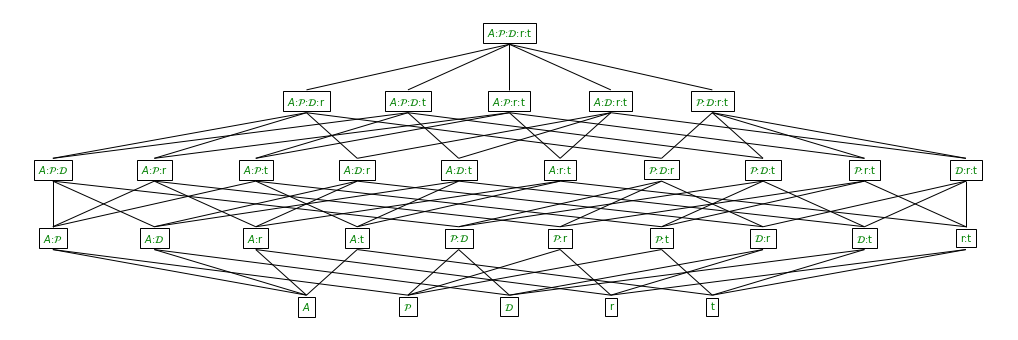lattice of parameter influence on accuracy: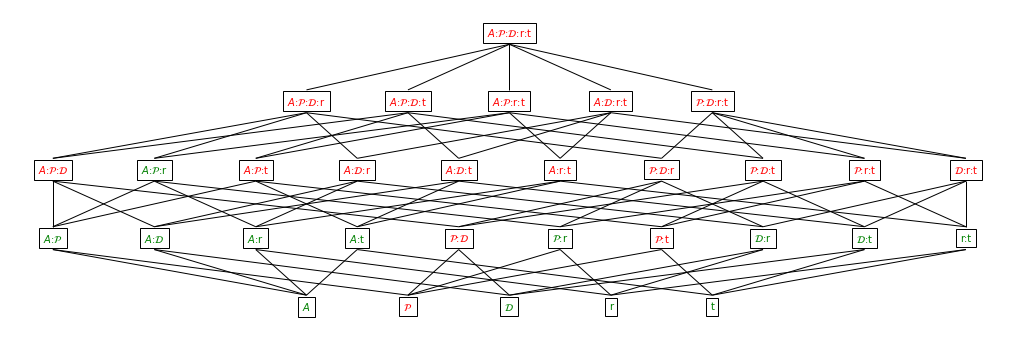lattice of parameter influence on distance: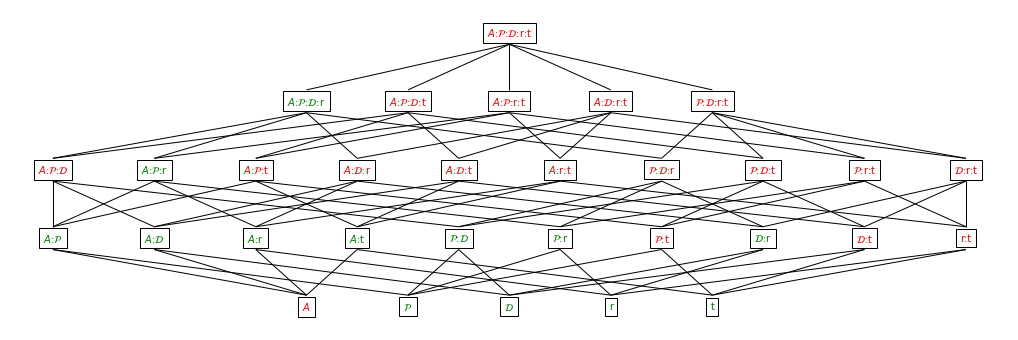### 2 variables interactions on accuracy¶

interaction of numberOfAgents with numberOfFeatures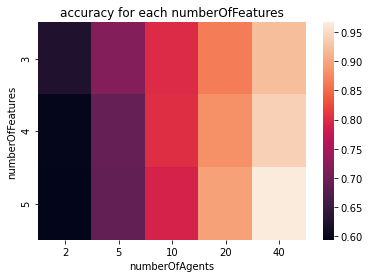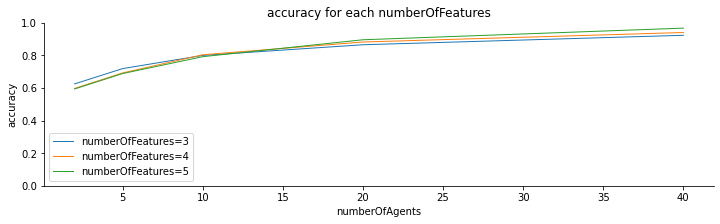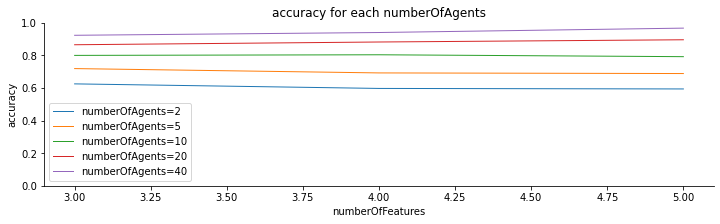interaction of numberOfAgents with numberOfClasses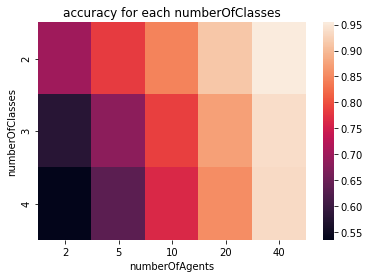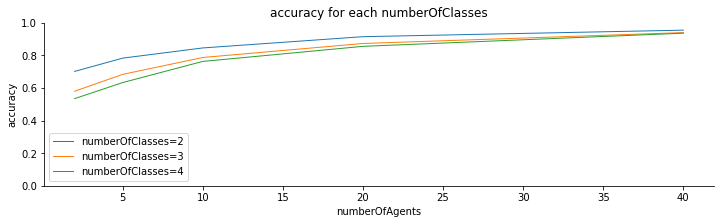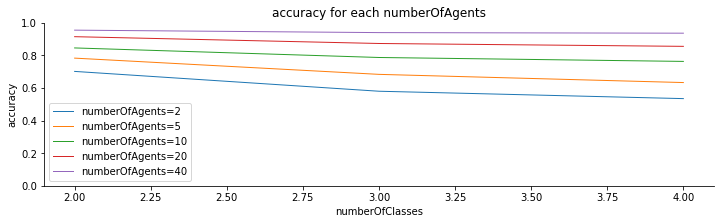interaction of numberOfAgents with ratio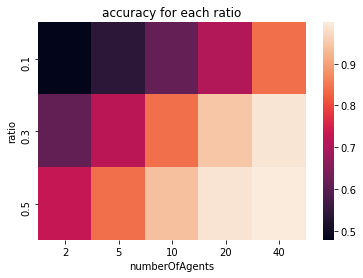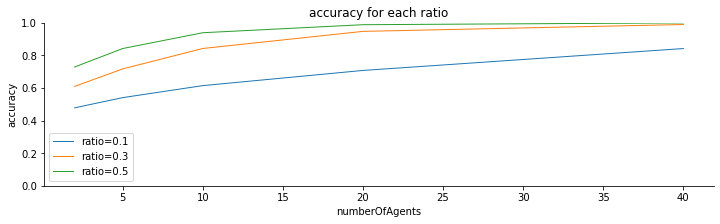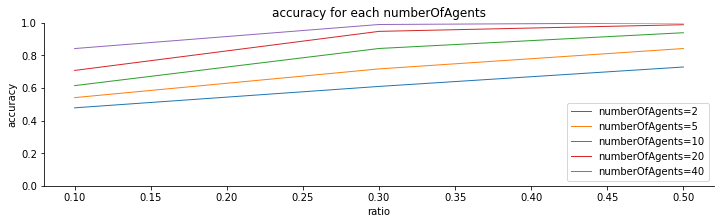interaction of numberOfAgents with sampleRatio